# Vanishing cycle

Letbe an-dimensional complex manifold with boundary,a Riemann surface and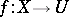a proper holomorphic mapping which has no critical points on the boundary ofand only non-degenerate critical points on the interior with distinct critical values. Letbe a path onsuch that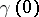is a critical value ofbutis a regular value for. For, write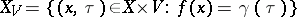. The group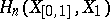is then infinite cyclic. An-chainongenerating it is called a Lefschetz thimble and its boundary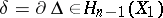a (Lefschetz) vanishing cycle [a1]. It is uniquely determined byup to sign. Two cases are of particular importance: the case of a Lefschetz pencil of hyperplane sections of a projective variety (see Monodromy transformation) and of semi-universal deformations of isolated complete intersection singularities [a2], [a3]. In the latter case, one first restricts the semi-universal deformation to a smooth curve which intersects the discriminant transversely. Suitable choices of paths connecting a regular valuewith the critical values lead to (strongly or weakly) distinguished bases of the vanishing homology group.
If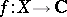is a holomorphic function on a complex spaceandis a constructible sheaf complex on, one obtains a constructible sheaf complexon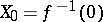in the following way. Let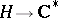be a universal covering and let,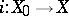be the natural mappings. Then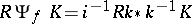. The functoris called the nearby cycle functor. There is a distinguished triangle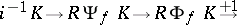in the derived category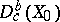. Hereis the vanishing cycle functor associated to[a4].
If the sheaf complexis perverse, the same holds for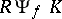and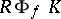. Ifis a complex manifold, by the Riemann–Hilbert correspondence one has vanishing and nearby cycle functors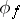and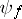in the category of regular holonomic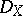-modules [a5], [a6] (see also-module; Derived category). They play a crucial role in the theory of mixed Hodge modules [a7].